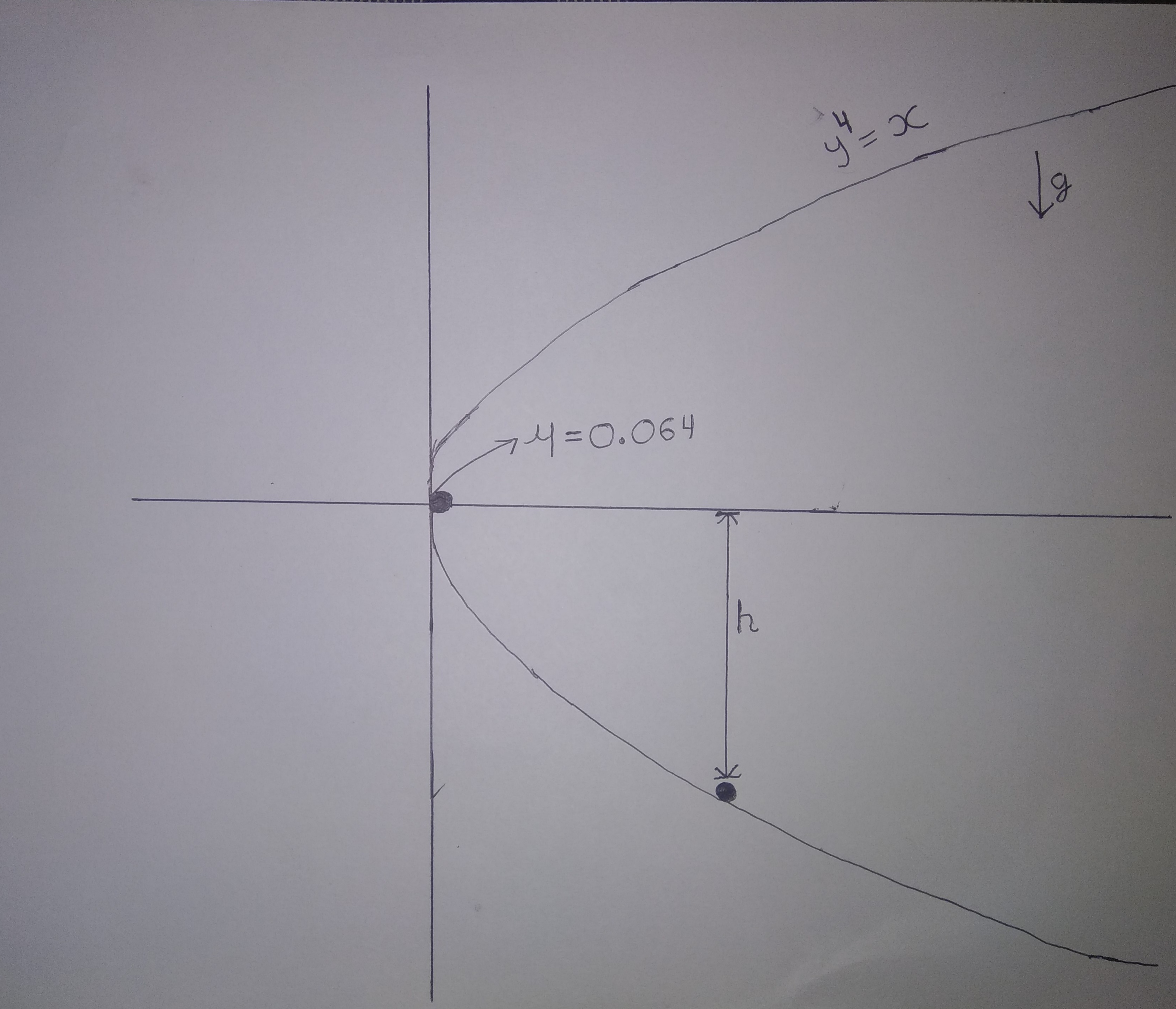# Graphical Mechanics (Part 2)A particle of mass $m$ is released from $\text{origin}$ on a $\text{rough wedge}$ shaped $x = y^4$. After falling for time $t$, it stops.

Enter value of $t$ in $\text{seconds}$

# Details and Assumptions

• Take acceleration due to gravity $\color{#3D99F6}{g = 10m/s^2}$

• Coefficient of friction $\color{#3D99F6}{\mu = 0.064}$

Inspiration Aniket Sanghi

Try similar problem by me

All of my problems are original

×